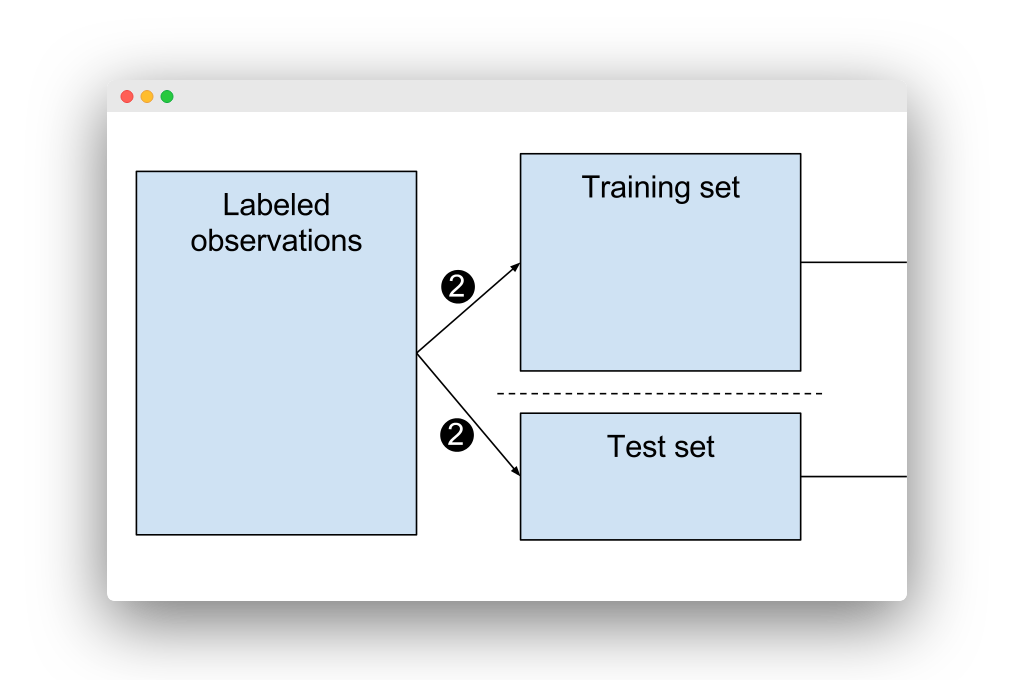Data is infinite. Data scientists have to deal with that every day!

Sometimes we have data, we have features and we want to try to predict what can happen.

To do that, data scientists put that data in a Machine Learning to create a Model.

Related course: Python Machine Learning Course

Let’s set an example:

1. A computer must decide if a photo contains a cat or dog.
2. The computer has a training phase and testing phase to learn how to do it.
3. Data scientists collect thousands of photos of cats and dogs.
4. That data must be split into training set and testing test.

Then is when split comes in.

## Train test split

### SplitKnowing that we can’t test over the same data we train, because the result will be suspicious… How we can know what percentage of data use to training and to test?

Easy, we have two datasets.

• One has independent features, called (x).
• One has dependent variables, called (y).

To split it, we do:

x Train – x Test / y Train – y Test

That’s a simple formula, right?

x Train and y Train become data for the machine learning, capable to create a model.

Once the model is created, input x Test and the output should be equal to y Test.

The more closely the model output is to y Test: the more accurate the model is.

Then split, lets take 33% for testing set (whats left for training).

You can verify you have two sets:

Data scientists can split the data for statistics and machine learning into two or three subsets.

• Two subsets will be training and testing.
• Three subsets will be training, validation and testing.

Anyways, scientists want to do predictions creating a model and testing the data.

When they do that, two things can happen: overfitting and underfitting.

### Overfitting

Overfitting is most common than Underfitting, but none should happen in order to avoid affect the predictability of the model.

So, what that means?

Overfitting can happen when the model is too complex.

Overfitting means that the model we trained has trained “too well” and fit too closely to the training dataset.

But if it’s too well, why there’s a problem? The problem is that the accuracy on the training data will unable accurate on untrained or new data.

To avoid it, the data can’t have many features/variables compared to the number of observations.

### Underfitting

Underfitting can happen when the model is too simple and means that the model does not fit the training data.

To avoid it, the data need enough predictors/independent variables.

Before, we’ve mentioned Validation.

If you are new to Machine Learning, then I highly recommend this book.

### Validation

Cross Validation is when scientists split the data into (k) subsets, and train on k-1 one of those subset.

The last subset is the one used for the test.

Some libraries are most common used to do training and testing.

• Pandas: used to load the data file as a Pandas data frame and analyze it.
• Sklearn: used to import the datasets module, load a sample dataset and run a linear regression.
• Matplotlib: using pyplot to plot graphs of the data.

Finally, if you need to split database, first avoid the Overfitting or Underfitting.

Do the training and testing phase (and cross validation if you want).

Use the libraries that suits better to the job needed.

Machine learning is here to help, but you have to how to use it well.